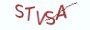① 质数是大于1的自然数

② 质数只能被1或它本身整除。

# 3.1.2 算法实现

```# __author__ = 薯条老师

import random

numbers = [random.randint(2,  10**4) for _ in range(10)]
print("大于1的10个随机数:{}".format(numbers))
max_2th_prime_number = max_prime_number = None

for number in numbers:
for _ in range(2, number):
if number % _ == 0:
break
else:
if max_prime_number is None:
max_prime_number = number
elif number > max_prime_number:
max_2th_prime_number = max_prime_number
max_prime_number = number
elif max_2th_prime_number is None or _ > max_2th_prime_number:
max_2th_prime_number = number
else:
print("最大质数:{}\n次大质数:{}".format(max_prime_number, max_2th_prime_number))```

# 3.1.3 最具实力的小班培训

`(1) Python后端工程师高薪就业班，月薪11K-18K，免费领取课程大纲(2) Python爬虫工程师高薪就业班，年薪十五万，免费领取课程大纲(3) Java后端开发工程师高薪就业班，月薪11K-20K, 免费领取课程大纲(4) Python大数据分析，量化投资就业班，月薪12K-25K,免费领取课程大纲`## 注册账号